# Class 8 RD Sharma Solutions – Chapter 3 Squares and Square Roots – Exercise 3.7 | Set 1

• Last Updated : 06 Apr, 2021

### Question 1) 84.8241

Solution: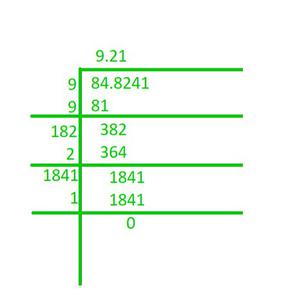Attention reader! All those who say programming isn't for kids, just haven't met the right mentors yet. Join the  Demo Class for First Step to Coding Coursespecifically designed for students of class 8 to 12.

The students will get to learn more about the world of programming in these free classes which will definitely help them in making a wise career choice in the future.

Hence, the square root of 84.8241 is 9.21.

### Question 2) 0.7225

Solution: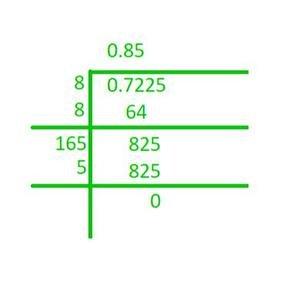Hence, the square root of 0.7225 is 0.85.

### Question 3) 0.813604

Solution: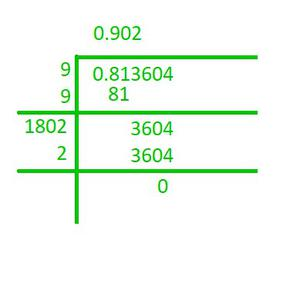Hence, the square root of 0.813604 is 0.902.

### Question 4) 0.00002025

Solution: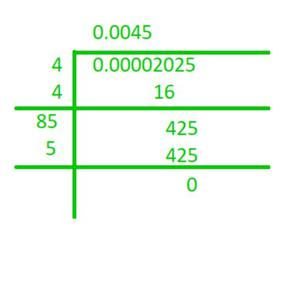Hence, the square root of 0.00002025 is 0.0045.

### Question 5) 150.0625

Solution: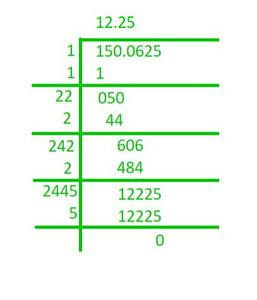Hence, the square root of 150.0625 is 12.25.

### Question 6) 225.6004

Solution: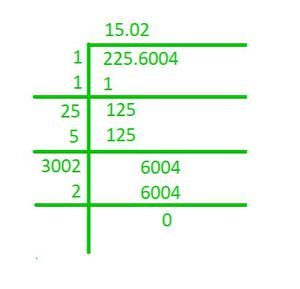Hence, the square root of 225.6004 is 15.02.

### Question 7) 3600.720036

Solution: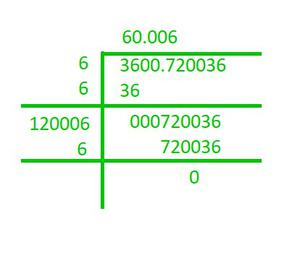Hence, the square root of 3600.720036 is 60.006.

### Question 8) 236.144689

Solution: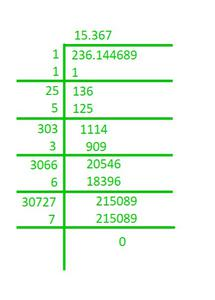Hence, the square root of 236.144689 is 15.367.

### Question 9) 0.00059049

Solution: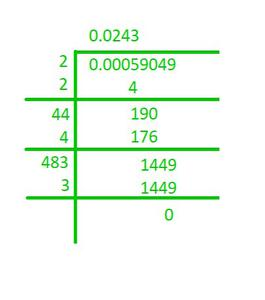Hence, the square root of 0.00059049 is 0.0243.

### Question 10) 176.252176

Solution:Hence, the square root of 176.252176 is 13.276.

### Chapter 3 Squares and Square Roots – Exercise 3.7 | Set  2

My Personal Notes arrow_drop_up